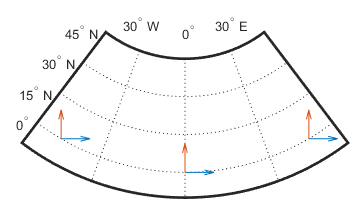# vinvtran

Transform direction on map to azimuth on ellipsoid

## Syntax

```az = vinvtran(x,y,th) az = vinvtran(mstruct,x,y,th) [az,len] = vinvtran(...) ```

## Description

`az = vinvtran(x,y,th) ` transforms an angle in the projection space at the point specified by `x` and `y` into an azimuth angle in geographic coordinates. The map projection currently displayed is used to define the projection space. The input angles must be in the same units as specified by the current map projection. The inputs can be scalars or matrices of equal size. The angle in the projection space angle `th` is defined as positive counterclockwise from the x-axis.

`az = vinvtran(mstruct,x,y,th) ` uses the map projection defined by the input `struct` to compute the map projection.

`[az,len] = vinvtran(...) ` also returns the vector length in the geographic coordinate system. A value of 1 indicates no scale distortion for that angle.

## Background

While vectors along the y-axis always point to north in a cylindrical projection in the normal aspect, they can point east or west of north on conic, azimuthal, and other projections. This function computes the geographic azimuth for angles in the projected space.

## Examples

Sample calculations:

```axesm('eqdconicstd','maplatlim',[-10 45],'maplonlim',[-55 55]) gridm; framem; mlabel; plabel; axis off mstruct = gcm; [x,y] = projfwd(mstruct,[0 0 0],[-45 0 45]); quiver(x,y,[0.2 0.2 0.2],[0 0 0],0) quiver(x,y,[0 0 0],[0.2 0.2 0.2],0)``````vinvtran(x,y,[0 0 0]) ```
```ans = 56.1765 90.0000 123.8235```
```vinvtran(x,y,[90 90 90]) ```
```ans = 332.8360 0.0000 27.1640```

## Limitations

This transformation is limited to the region specified by the frame limits in the current map definition.

## Tips

The geographic azimuth angle is measured clockwise from north. The projection space angle is measured counterclockwise from the x-axis.

This function uses a finite difference technique. The geographic coordinates are perturbed slightly in different directions and projected. A small amount of error is introduced by numerical computation of derivatives and the variation of map distortion parameters.

## Version History

Introduced before R2006a